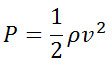# AF-Pressure

By | 9 December, 2018
Dynamic pressure:where
– P : Dynamic pressure (N/m2 (Pa), lbf/ft2 (psf))
– ρ : (kg/m3)
– v : Velocity (m/s, ft/s)

Pressure drop:– ƒ : Friction Factor
– L : Length of Pipe (m)
– D: Pipe Diameter (m)
– ρ : (kg/m3)
– v : Velocity (m/s)
Pressure Calculator
 Density (ρ): [kg/m3] Velocity (v): [m/s] Internal Diameter (D): [mm] Length of Pipe (L): [m] Friction Factor (ƒ): Dynamic Pressure (p): [Pa] Pressure drop (Δp): [Pa]